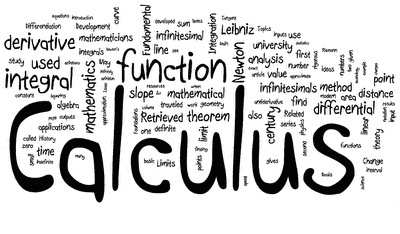Pre-CalculusCOURSE DESCRIPTIONThis course is designed to cover topics in Algebra ranging from polynomial, rational, and exponential functions to conic sections. Trigonometry concepts such as Law of Sines and Cosines will be introduced. Students will then begin analytic geometry and calculus concepts such as limits, derivatives, and integrals. This class is important for any student planning to take a college algebra or college pre-calculus class.

CHAPTERSModule 1: Functions
1.1: Functions and Function Notation
1.2 Domain and Range
1.3 Rates of Change and Behavior of Graphs
1.4 Composition of Functions
1.5 Transformation of Functions
1.6 Inverse Functions

Module 2: Linear Function
2.1 Linear Functions
2.2 Graphs of Linear Functions
2.3 Modeling with Linear Functions
2.4 Fitting Linear Models to Data
2.5 Absolute Value Functions

Module 3: Polynomial and Rational Functions
3.1 Power Functions & Polynomial Functions
3.3 Graphs of Polynomial Functions
3.4 Rational Functions

Module 4: Exponential and Logarithmic Functions
4.1 Exponential Functions
4.2 Graphs of Exponential Functions
4.3 Logarithmic Functions
4.4 Logarithmic Properties
4.5 Graphs of Logarithmic Functions
4.6 Exponential and Logarithmic Models
4.7 Fitting Exponentials to Data

Module 5: Trigonometric Functions of Angles
5.1 Circles
5.2 Angles
5.3 Points on Circles using Sine and Cosine
5.4 The Other Trigonometric Functions
5.5 Right Triangle TrigonometryModule

Module 6: Periodic Functions
6.1 Sinusoidal Graphs
6.2 Graphs of the Other Trig Functions
6.3 Inverse Trig Functions
6.4 Solving Trig Equations
6.5 Modeling with Trigonometric Equations

Module 7: Trigonometric Equations and Identities
7.1 Solving Trigonometric Equations with Identities
7.3 Double Angle Identities
7.4 Modeling Changing Amplitude and Midline

Module 8: Further Applications of Trigonometry
8.1 Non-right Triangles: Law of Sines and Cosines
8.2 Polar Coordinates
8.3 Polar Form of Complex Numbers
8.4 Vectors
8.5 Parametric Equations

﻿
QQ咨询